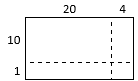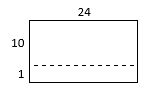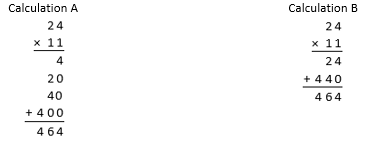# Multi-Digit Multiplication

## Objective

Multiply two-digit by two-digit numbers using two partial products and assess the reasonableness of the product.

## Common Core Standards

### Core Standards

?

• 4.NBT.B.5 — Multiply a whole number of up to four digits by a one-digit whole number, and multiply two two-digit numbers, using strategies based on place value and the properties of operations. Illustrate and explain the calculation by using equations, rectangular arrays, and/or area models.

?

• 3.NBT.A.3

• 4.NBT.A.1

• 4.NBT.B.4

• 3.OA.B.5

• 3.OA.C.7

## Criteria for Success

?

1. Understand that products can be computed by decomposing numbers into base-ten units, finding partial products of these base-ten units, then adding these partial products together based on the distributive property (e.g., 36 x 54 = (36 x 50) + (36 x 4)). (Note that students need not know the term “distributive property.”)
2. Multiply a two-digit whole number by a two-digit whole number using area models and the standard algorithm.
3. Assess the reasonableness of answers using mental computation and estimation strategies including rounding (MP.1).
4. Solve one-step word problems involving multiplication of two-digit by two-digit numbers (on the Problem Set and Homework) (MP.4).

## Tips for Teachers

?

#### Remote Learning Guidance

If you need to adapt or shorten this lesson for remote learning, we suggest prioritizing Anchor Task 1 (benefits from discussion), Anchor Task 2 (benefits from worked example), and Anchor Task 3 (can be done independently). Find more guidance on adapting our math curriculum for remote learning here.

#### Fishtank Plus

• Problem Set
• Student Handout Editor
• Vocabulary Package

?

### Problem 1

Joy started to solve the math problem 24 x 11 with the area model. Her work looked like this:But, her classmate Sophia told her that instead of using four areas, she could solve it using only two and drew the area model below:1. How are Joy’s and Sophia’s area models similar?
2. How are Joy’s and Sophia’s area models different?
3. Do you think Sophia’s method will work? Why or why not?

#### References

Open Up Resources Grade 6 Unit 5 Lesson 7 (Teacher Version)Activity 2

Modified by The Match Foundation, Inc.
EngageNY Mathematics Grade 4 Mathematics > Module 3 > Topic H > Lesson 37Concept Development

Grade 4 Mathematics > Module 3 > Topic H > Lesson 37 of the New York State Common Core Mathematics Curriculum from EngageNY and Great Minds. © 2015 Great Minds. Licensed by EngageNY of the New York State Education Department under the CC BY-NC-SA 3.0 US license. Accessed Dec. 2, 2016, 5:15 p.m..

Modified by The Match Foundation, Inc.

### Problem 2

Joy and Sophia wrote the partial products from their area models as follows:1. Which two numbers are being multiplied to get 4 in Calculation A? Which numbers are being multiplied to get 24 in Calculation B? How are the other partial products below calculated?
2. Which calculation corresponds with Joy’s area model from Anchor Task #1? With Sophia’s area model?

#### References

EngageNY Mathematics Grade 4 Mathematics > Module 3 > Topic H > Lesson 37Concept Development

Grade 4 Mathematics > Module 3 > Topic H > Lesson 37 of the New York State Common Core Mathematics Curriculum from EngageNY and Great Minds. © 2015 Great Minds. Licensed by EngageNY of the New York State Education Department under the CC BY-NC-SA 3.0 US license. Accessed Dec. 2, 2016, 5:15 p.m..

Modified by The Match Foundation, Inc.
Open Up Resources Grade 6 Unit 5 Lesson 7 (Teacher Version)Activity 2

Modified by The Match Foundation, Inc.

### Problem 3

Use the standard algorithm to find the following products. Then assess the reasonableness of your answer.

1.    42 x 22
2.    35 x 26
3.    24 x 36
4.    56 x 63

#### References

EngageNY Mathematics Grade 4 Mathematics > Module 3 > Topic H > Lesson 37Concept Development

Grade 4 Mathematics > Module 3 > Topic H > Lesson 37 of the New York State Common Core Mathematics Curriculum from EngageNY and Great Minds. © 2015 Great Minds. Licensed by EngageNY of the New York State Education Department under the CC BY-NC-SA 3.0 US license. Accessed Dec. 2, 2016, 5:15 p.m..

Modified by The Match Foundation, Inc.

## Problem Set & Homework

#### Discussion of Problem Set

• Look at #2c and #2d. What do you notice about their factors? What do you notice about their products?
• How did you determine the total amount of money the store made in #4? How did you round the total? How is that different from the way we’ve rounded in the unit thus far?
• Look at #6c and #6d. What do you notice about their factors? What do you notice about their products?
• Did you use any strategies from Lesson 18 to solve any problems on today’s Problem Set? For example, #6a or #6c?

?

### Problem 1

Which method can be used to solve 11 x 13?

A. Multiply 11 x 10 and 10 x 3, then add the two products.

B. Multiply 11 x 10 and 11 x 3, then add the two products.

C. Multiply 11 x 100 and 10 x 3, then add the two products.

D. Multiply 11 x 100 and 11 x 3, then add the two products.

#### References

Question #40

From EngageNY.org of the New York State Education Department. New York State Testing Program Grade 4 Common Core Mathematics Test Released Questions June 2017. Internet. Available from https://www.engageny.org/resource/released-2017-3-8-ela-and-mathematics-state-test-questions/file/150261; accessed March 7, 2018, 1:59 p.m..

### Problem 2

 a.   72 x 43 b.  35 x 53

#### References

EngageNY Mathematics Grade 4 Mathematics > Module 3 > Topic H > Lesson 38Exit Ticket, Questions #1 and #2

Grade 4 Mathematics > Module 3 > Topic H > Lesson 38 of the New York State Common Core Mathematics Curriculum from EngageNY and Great Minds. © 2015 Great Minds. Licensed by EngageNY of the New York State Education Department under the CC BY-NC-SA 3.0 US license. Accessed Dec. 2, 2016, 5:15 p.m..

Modified by The Match Foundation, Inc.

### Mastery Response

?